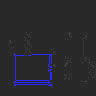## Selasa, 07 Juli 2009

### Jawaban Tugas 4

Source codex :
bool CxImage::Jitter(long radius)
{
// check if the image is valid, this should be always the first line in
// the function
if (!pDib) return false;

// local variables
long nx,ny;

// temporary image to store the partial results of the algorithm
CxImage tmp(*this,pSelection!=0,true,true);

// limit the effects of the functions only in the smallest rectangle that
// holds the selected region (defined with the Selection...() functions ),
// this will speed up the loops.
long xmin,xmax,ymin,ymax;
if (pSelection){
xmin = info.rSelectionBox.left; xmax = info.rSelectionBox.right;
ymin = info.rSelectionBox.bottom; ymax = info.rSelectionBox.top;
} else {
xmin = ymin = 0;
xmax = head.biWidth; ymax=head.biHeight;
}

// main loop : scan the image in vertical direction
for(long y=ymin; y

// monitor the progress of the loops
info.nProgress = (long)(100*y/head.biHeight);

// let the application a way to exit quickly
if (info.nEscape) break;

// main loop : scan the image in horizontal direction
for(long x=xmin; x

// if the feature is enabled, process only the pixels inside the
// selected region
#if CXIMAGE_SUPPORT_SELECTION
if (SelectionIsInside(x,y))
#endif //CXIMAGE_SUPPORT_SELECTION
{
// main algorithm
nx=x+(long)((rand()/(float)RAND_MAX - 0.5)*(radius*2));
ny=y+(long)((rand()/(float)RAND_MAX - 0.5)*(radius*2));
if (!IsInside(nx,ny)) {
nx=x;
ny=y;
}

// save the result in the temporary image.
// if you can, use PixelColor only for 24 bpp images,
// and PixelIndex for 8, 4 and 1 bpp images : it's faster
if (head.biClrUsed==0){
tmp.SetPixelColor(x,y,GetPixelColor(nx,ny));
} else {
tmp.SetPixelIndex(x,y,GetPixelIndex(nx,ny));
}

// if the feature is enabled, process also the pixels
// in the alpha layer
#if CXIMAGE_SUPPORT_ALPHA
tmp.AlphaSet(x,y,AlphaGet(nx,ny));
#endif //CXIMAGE_SUPPORT_ALPHA

}
}

}

// save the result and exit
Transfer(tmp);
return tru
e;
}